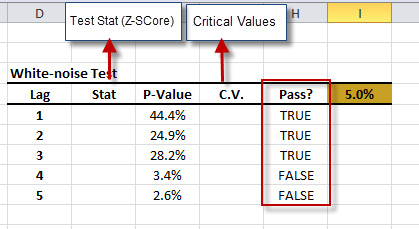# White-noise Test

To examine a given time series for signs of serial correlation, we conduct a statistical test for white noise (Ljung-Box Test):

$$H_{o}: \rho_{1}=\rho_{2}=...=\rho_{m}=0$$

$$H_{1}: \exists \rho_{k}\neq 0$$

Where $\rho_i$ is the autocorrelation function for the i-th lag. The selection of $m$ (max. lags) is rather arbitrary, but experience shows that we achieve the best results with $m=\left \lceil \ln(N) \right \rceil$ as the input sample size.

NumXL provides an intuitive interface to help Excel users conduct a white noise test using several lag orders. In this tutorial, we’ll demonstrate the steps to perform a thorough white noise test using NumXL functions and wizards in Excel.

## Process

1. Select an empty cell to store the histogram table.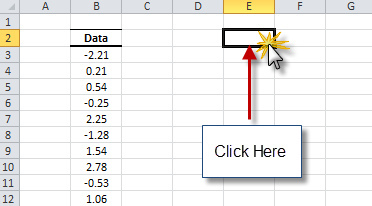2. Locate the Statistical Test (STAT TEST) icon in the toolbar (or menu in Excel 2003) and click on the down-arrow. When the drop-down menu appears, select the “White Noise Test”.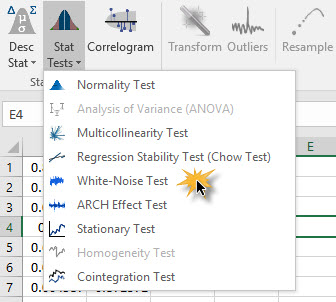3. The white noise dialog box appears.
4. Select the cell range for the input data.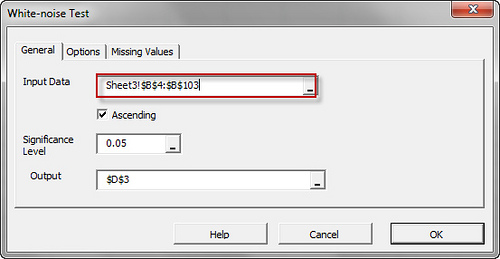5. Click the “Options” Tab. Select the maximum lag order in the test (i.e. m).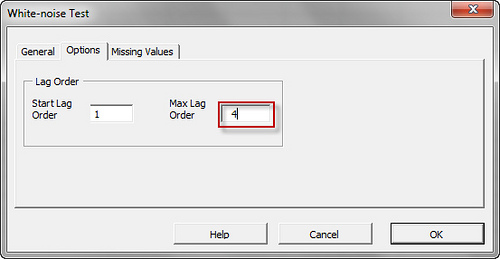6. If your data include one or more intermediate observations with missing values, click the “Missing Values” tab.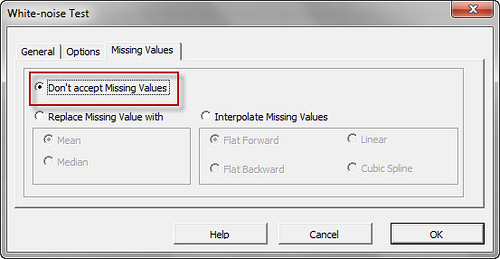7. Click “OK”.

## Output

The white noise wizard generates white noise statistics for different lags scenarios.